# JIPMAT Question Paper 2021 | JIPMAT DILR

###### JIPMAT Question Paper | JIPMAT DILR Question Paper

JIPMAT Question Paper DILR. Solve questions from JIPMAT 2021 Question Paper and check the solutions to get adequate practice.

1. #### JIPMAT 2021 Question Paper - DILR

In this question, a number series is given with one term missing. Choose the correct option that will continue the same pattern. 17, 19, 15, 23, 7, 39, ?

1. 18
2. -25
3. 25
4. 9

2. #### JIPMAT 2021 Question Paper - DILR

In this question, an alphabet series is given with one term missing. Choose the correct option that will continue the same pattern. MOQ, SUW, YAC, ?

1. DHJ
2. EGI
3. FIJ

3. #### JIPMAT 2021 Question Paper - DILR

In this question, a letter series is given where some of the letters are missing. The missing letters are given in the order as one of the options below it. Choose the correct option. a ________ c ________ ba ________ ca ________ cb

1. abcc
2. acba
3. bcaa
4. bcba

4. #### JIPMAT 2021 Question Paper - DILR

There is a certain relation between two given numbers on one side of : : and one number is given on another side of : : . A number is to be found from the given options having the same relation with this number as the given pair. 210 : 380 : : 182 : ?

1. 156
2. 240
3. 272
4. 342

5. #### JIPMAT 2021 Question Paper - DILR

There is a certain relation between two given words on one side of : : and one word is given on another side of : : . A word is to be found from the given options having the same relation with this word as the given pair.
Drill : Bore : : Sieve : ?

1. Pry
2. Rinse
3. Sift
4. Thresh

6. #### JIPMAT 2021 Question Paper - DILR

In this question, there are four options. Three of them are alike and one is different. Find the odd one out.

1. December, 2021
2. February, 2021
3. March, 2021
4. November, 2021

7. #### JIPMAT 2021 Question Paper - DILR

This question is based on the information given below : The six faces of cube are painted in a manner that no two adjacent faces have the same colour. The three colours used in painting are red, blue and green. The cube is then cut into 36 smaller cubes in a manner that 32 cubes are of one size and the rest of a bigger size and each of the bigger cube has no red side. How many cubes only have one side coloured?

1. 0
2. 16
3. 4
4. 8

8. #### JIPMAT 2021 Question Paper - DILR

Which one word cannot be made from the word ‘MIRACULOUS’?

1. LOCUS
2. MOLAR
3. SCAR
4. SOLACE

9. #### JIPMAT 2021 Question Paper - DILR

If ‘+’ means ‘÷’, ‘–’ means ‘×’, ‘÷’ means ‘+’ and ‘×’ means ‘–’ then 36 × 12 + 4 ÷ 6 + 2 – 3 is equal to

1. 62. 18
3. 2
4. 42

10. #### JIPMAT 2021 Question Paper - DILR

In this question, relationship between different elements is shown in the statements. The statements are followed by two conclusions numbered I and II.
Statement : H > P ≤ E < L = R < V ; F ≥ V
Conclusion : I. F > E
II. H > R

1. If both the conclusions I and II are true
2. If neither conclusion I nor conclusion II is true
3. If only conclusion I is true
4. If only conclusion II is true

11. #### JIPMAT 2021 Question Paper - DILR

Study the information given below and answer the question that follow. There are four friends A, B, C, D. One of them is a cricketer and studies chemistry and biology. A and B play football. Both football players study mathematics. D is a boxer. One football player also studies physics. The boxer studies mathematics and accounts. All the friends study two subjects each and play one game each. How many games are played and subjects studied by the friends A, B, C, D?

1. 2 games and 3 subjects
2. 2 games and 4 subjects
3. 3 games and 4 subjects
4. 3 games and 5 subjects

12. #### JIPMAT 2021 Question Paper - DILR

(i) Q is brother of R.
(ii) P is sister of Q
(iii) T is brother of S
(iv) S is daughter of R
Who are the niece of Q?

1. P and T
2. Q and T
3. R and P
4. S and T

13. #### JIPMAT 2021 Question Paper - DILR

In a certain code, ‘il be pee’ means ‘roses are blue’ , ‘sik hee’ means ‘red flowers’ and ‘pee mit hee’ means ‘flowers are vegetables’, then which word stands for ‘red’?

1. be
2. cannot be determined
3. hee
4. sik

14. #### JIPMAT 2021 Question Paper - DILR

Sandhya walks 10 m towards the south. Turning to the left, she walks 20 m and then turns to her right. After moving a distance of 20 m, she turns to the right and walks 20 m. Finally, she turns to the right and moves 10 m. In which direction is she moving

1. East
2. North
3. South
4. West

15. #### JIPMAT 2021 Question Paper - DILR

Which of the following figures correctly represent the relation between Tall men, Black haired people, Indians

1.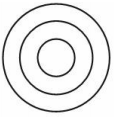2.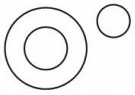3.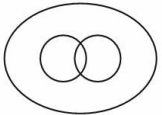4.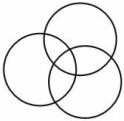16. #### JIPMAT 2021 Question Paper - DILR

Chandrika is 8 ranks ahead of Jaya who ranks twenty sixth in a class of 42. What is Chandrika’s rank from the last?

1. 24th
2. 25th
3. 34th
4. 9th

17. #### JIPMAT 2021 Question Paper - DILR

X was born on March 6, 2014. The same year Independence Day was celebrated on Friday. On which day X was born?

1. Friday
2. Saturday
3. Thursday
4. Wednesday

18. #### JIPMAT 2021 Question Paper - DILR

In this question, find out how many such pairs of letters are there in the given word each of which has as many letters between them in the word as in the English alphabet.
CREATIVE

1. Four
2. One
3. Three
4. Two

19. #### JIPMAT 2021 Question Paper - DILR

Study the following arrangement carefully and answer the question given below.
A B 7 C D 9 Z Y * P Z M © K S 3 ⇑ 5 N T @
How many such digits are there in the sequence each of which is immediately preceded as well as followed by a letter?

1. None
2. One
3. Three
4. Two

20. #### JIPMAT 2021 Question Paper - DILR

In this question, arrange the given words in a meaningful sequence and then choose the most appropriate sequence from amongst the options provided below the question.
A. Presentation
B. Recommendation
C. Arrival
D. Discussion
E. Introduction

1. C, E, A, D, B
2. C, E, D, B, A
3. C, E, D, B, A
4. E, C, D, A, B

21. #### JIPMAT 2021 Question Paper - DILR

Below a question is given along with two statements numbered I and II. You have to decide whether the data provided in the statements are sufficient to answer the question.
If a and b are integers, is (a + b) an odd number?
Statement I : 8 < a < 11
Statement II : 7 < b < 10
Choose you answer from the options given below:

1. If the data in statement I alone are sufficient to answer the question.
2. If the data in statement I and statement II both are necessary to answer the question
3. If the data in statement I and statement II together are not sufficient to answer the question.
4. If the data in statement II alone are sufficient to answer the question.

22. #### JIPMAT 2021 Question Paper - DILR

Study the graph and answer the question that follows:
The graph indicates the cost of the production of a firm.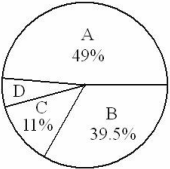A = Raw material cost
B = Packing material cost
C = Fixed manufacturing expenses
D = Labour cost
The total value in rupees of all sectors is 120.0 lakh. If the total labour cost increases to Rs. 2.4 lakh, what percentage increase does it represent?

1. 25%
2. 300%
3. 400%
4. 75%

23. #### JIPMAT 2021 Question Paper - DILR

In this question, there are two statements followed by two conclusions numbered I and II. You have to take the given statement to be true, even if it seems to be at variance with commonly known facts and then decide which of the conclusions logically follow(s) from the given statements.
Statement : No women can vote.
Some women are politicians.
Conclusion : I. Male politicians can vote.
II. Some politicians can vote.

1. If both conclusion I and II follow.
2. If neither conclusion I nor conclusion II follows
3. If only conclusion I follows
4. If only conclusion II follows

24. #### JIPMAT 2021 Question Paper - DILR

In this question, a statement is given which is followed by two conclusions numbered I and II. You have to assume everything in the statement to be true, then consider the conclusions and decide which of them logically follow(s).
Statement : Fortune favours the brave.
Conclusion : I. Risks are necessary for success.
II. Cowards die many times before their death.

1. If both conclusion I and II follow
2. If neither conclusion I nor conclusion II follows
3. If only conclusion I follows
4. If only conclusion II follows

25. #### JIPMAT 2021 Question Paper - DILR

In this question, there are two statements labelled as Assertive (A) and Reason (R). Assertion (A) : An electric bulb makes a ‘bang’ when it is broken.
Reason (R) : The air inside the bulb rushes out immediately on breaking.

1. A is false but R is true.
2. A is true but R is false.
3. Both A and R are true and R is the correct explanation of A.
4. Both A and R are true but R is not the correct explanation of A.

26. #### JIPMAT 2021 Question Paper - DILR

In a family, each daughter has the same number of brothers as she has sisters and each son has twice as many sisters as he has brothers. How many sons are there in the family?

1. 2
2. 3
3. 4
4. 5

27. #### JIPMAT 2021 Question Paper - DILR

The diagram indicates the number of students taking different subjects. Which subject was taken by the largest number of students?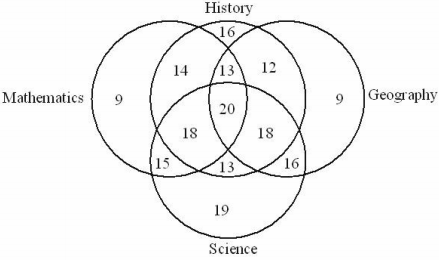1. Geography
2. History
3. Mathematics
4. Science

28. #### JIPMAT 2021 Question Paper - DILR

Study the following information and answer the question.
Seven villages A, B, C, D, E, F and G are situated as follows:
E is 2 km to the west of B
F is 2 km to the north of A
C is 1 km to the west of A
D is 2 km to the south of G
G is 2 km to the east of C
D is exactly in the middle of B and E.
How far is D from F (in km) as the crow flies?

1. √5
2. 2 √2
3. √17
4. √20

29. #### JIPMAT 2021 Question Paper - DILR

In this question, choose that set of numbers from the four options, that is similar to the set (63, 49, 35)

1. (64, 40, 28)
2. (72, 40, 24)
3. (72, 48, 24)
4. (81, 63, 45)

30. #### JIPMAT 2021 Question Paper - DILR

In this question, a group three interrelated words are given. You have to study the relationship between these words and choose another group with similar relationship from the given options. Pink : Red : White

1. Brown : Black : Blue
2. Green : Blue : Yellow
3. Orange : Yellow : Black
4. Yellow : Red : Green

31. #### JIPMAT 2021 Question Paper - DILR

The question consists of two words that have a certain relationship to each other, followed by four lettered pairs of words. Select the lettered pair that has the same relationship as the original pair of words.
Wind : Gale

1. Disaster : Calamity
2. Disgust : Infatuation
3. Love : Passion
4. Storm : Sea

32. #### JIPMAT 2021 Question Paper - DILR

In this question, four numbers are given. Out of these, three are alike in a certain way and one is different. Choose the one which is different from the rest three.

1. 5698
2. 7894
3. 8793
4. 9865

33. #### JIPMAT 2021 Question Paper - DILR

In this question, four groups of letters are given, out of which three are alike in a certain way while one is different. Choose the odd one.

1. B d E g
2. K m N p
3. P r S u
4. T w X z

##### Where is 2IIM located?

2IIM Online CAT Coaching
A Fermat Education Initiative,
58/16, Indira Gandhi Street,
Kaveri Rangan Nagar, Saligramam, Chennai 600 093

##### How to reach 2IIM?

Phone: (91) 44 4505 8484
Mobile: (91) 99626 48484 / 94459 38484
WhatsApp: WhatsApp Now
Email: info@2iim.com